# Analysis of a Hydration Calculator (Part 1)

Let’s consider a tool that will help bakers calculate the hydration of their baked goods. Let’s first consider my own needs, because I’m also a part of the target audience. I primarily bake sourdough bread, so let’s start there.

When I bake bread, I usually start with some amount of flour and some dry additives like salt, guar gum, or gluten. I don’t follow a recipe so this amount can vary between batches. From there I have to calculate the amount of water and sourdough starter I need depending on the dough hydration that I want. I usually calculate hydration based solely on the water component, and I don’t factor in the water and flour contributed by the starter. However, I do add quite a bit of starter to my sourdough because I don’t discard excess sourdough starter or constantly feed it. So it would probably be beneficial for me to include it in the calculation since it could easily increase the hydration by a few percentage points.

So in this case, we start with three known variables: dry ingredients, a target hydration, and some amount of sourdough starter, which is a 1 to 1 ratio of flour and water. And the unknown is the water to get to the hydration I want.

I could build something like this…

``````<!--HTML Element -->
<hydration-calculator calc="dry,hydration,starter=water"/>

<!-- Form -->
Dry: [ ]
Hydration: [ ]
Starter: [ ]
Water: Number``````

Which would calculate the water using the equation below, where S is the weight of the starter, H is the target hydration ranging from 0 to 1, and F is the dry weight.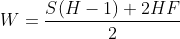And I think that would suit my current needs. But my other thought is that I can improve my own process. If I knew the exact weight I needed to fill 2 bread pans, I could start with that weight as one of my known variables. If I knew total weight, then I could calculate dry ingredients and water based on the total weight, the target hydration, and the amount of sourdough starter I had.

``````<!--HTML Element -->
<hydration-calculator calc="total,hydration,starter=flour,water"/>

<!-- Form -->
Total Weight: [ ]
Hydration: [ ]
Starter: [ ]
Flour: Number
Water: Number``````

And I could calculate dry and wet weight with the equation below, where F is the dry weight, T is the total weight, H is the target hydration, and W is the water weight.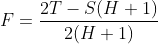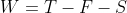If you’d like to derive these formulas yourself you can start from these fundamentals equations…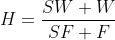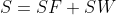Hydration is the total wet weight divided by the total dry weight. Starter weight is the starter flour plus the starter water. The total weight is the starter weight plus dry ingredients plus water.

In future posts, I’ll consider other users of the calculator and how we might change the user interface for those users. I’ll also consider how the different calculators could be implemented with a reusable HTML component and how we can improve the ergonomics specifically for developers.